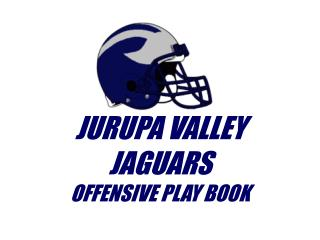Download PresentationJURUPA VALLEY JAGUARS

# JURUPA VALLEY JAGUARS - PowerPoint PPT PresentationDownload Presentation## JURUPA VALLEY JAGUARS

- - - - - - - - - - - - - - - - - - - - - - - - - - - E N D - - - - - - - - - - - - - - - - - - - - - - - - - - -
##### Presentation Transcript

1. JURUPA VALLEY JAGUARS OFFENSIVE PLAY BOOK

2. C T G G T X Y Q Z F H POSITIONS X

3. 1 4 2 3 RUNNINGBACK IDENTIFICATION X • QUARTERBACK • FULLBACK • HALFBACK • WINGBACK

4. 0 3 11 2 4 6 8 7 5 HOLE IDENTIFICATION X

5. C T G G T X Y Q Z F H SPLITS AND ALIGNMENTS X 2FT 2FT 2FT 2FT 3FT 7-12 YDS 2 YDS 3 YDS 4 YDS

6. RIGHT X O O X O O Y O Z H F LEFT Y O O X O O X Z O F H FORMATIONSRIGHT/LEFT RULES: Y TO the Call Z TO the Call X AWAY from Call H AWAY from Call

7. FORMATIONS RED/BLUE RED X O O X O O Y H O Z F BLUE Y O O X O O X Z O H F RULES (same as Right/Left except for H) Y TO the Call Z TO the Call X AWAY from Y H AWAY from Y and UP as Wing

8. FORMATIONS HEAVY RIGHT/HEAVY LEFT HEAVY RIGHT X O O X O O Y O Z F H HEAVY LEFT Y O O X O O X Z O H F RULES (same as Right/Left except for H) Y TO the Call Z TO the Call X AWAY from Y H TO the Y

9. FORMATIONS LIGHT RIGHT/LIGHT LEFT LIGHT LEFT Y O O X O O X O Z F H LIGHT RIGHT X O O X O O Y Z O H F RULES (same as Right/Left except for W) Y TO the Call Z AWAY from the Call X AWAY from Y H AWAY from Y

10. FORMATIONS FLIP RIGHT/FLIP LEFT LEFT Y O O X O O X O Z H F RIGHT X O O X O O Y Z O F H RULES (SAME AS HEAVY + LIGHT) Y TO the Call Z AWAY from Y X AWAY from Y H TOY

11. Split End (X) and TE (Y) Alignments OVER– X and Y on Same Side O O C O O Y X LOOSE– Y Split as WR X O O C O O Y TIGHT– X Lined up as TE X O O C O O Y TACK– Y Next to G X T T G C G Y

12. Split End (X) Alignments OVER OVER– X and Y on Same Side O O C O O Y X OVER RIGHT O O C O O Y X O Z H F OVER RED O O C O O Y X HO Z F OVER FLIP RIGHT O O C O O Y X Z O F H

13. Split End (X) and TE (Y) AlignmentsTIGHT TIGHT– X Lined up as TE X O O C O O Y

14. TE (Y) AlignmentsLOOSE LOOSE– Y Split as WR X O O C O O Y LOOSE RIGHT X O O C O O Y O Z H F LOOSE RED X O O C O O Y H O Z F

15. Halfback (H) and Wingback (Z) Alignmentsw/ Right Formation PRO– Z Lined Up as WR (Pro Right) X O O X O O Y O Z H F TWINS– Z splits T and X (Twins Right) X O O X O O Y Z O H F SLOT– H splits T and X (SLOT RIGHT) X O O X O O Y H O Z F TRIPS– X, Z & H away from Y (TRIPS RT) X O O X O O Y Z H O F OPEN– Pro and Slot Combo X O O X O O Y H O Z F TREY– H and Z TO Y (Trey Right) X O O X O O Y O HZ F BUNCH X O O X O O Y O HZ F SPREAD X O O X O O Y H O Z F

16. C T G G T X Y Q H Z F H MOTIONSH/HALFBACK MOTIONS FLY HIM HER HALF ROCKET

17. C T G G T X Y Q Z F H MOTIONSZ/WINGBACK MOTIONS JET ZAC ZOOM ZAP

18. 20 TRAP F C C C T G G T X Y B B B Q \$ Z T N T E F H 22 TRAP BUCK

19. 36 Sweep F C C C T G G T X Y B B B Q \$ Z T N T E F H 36 Sweep BUCK

20. OFFENSE VS. 50 F C C C T G G T X Y B B B Q \$ Z T N T E F H PLAY SERIES

21. OFFENSE VS. 53 F C C C T G G T X Y B B B Q B Z T N T E F H PLAY SERIES

22. OFFENSE VS. 53EAGLE F C C C T G G T X Y B B B Q B Z T N T E F H PLAY SERIES

23. OFFENSE VS. 44 F C C B B B B E T T E PLAY SERIES C T G G T X Y Q Z F H

24. OFFENSE VS. 43 F C C B B \$ B E T T E PLAY SERIES C T G G T X Y Q Z F H